# Half Wave Rectifier

## Half Wave Rectifier

The half wave rectifier conducts current only during the positive half cycles of the input supply voltage.

The negative half cycles  of the a.c. supply are suppressed. So no current is conducted and hence no voltage appears across the load. So current always flows in one direction (i.e. d.c. ) through the load.

### Circuit Connections of Half wave Rectifier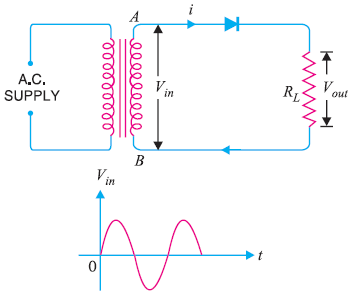Fig(1)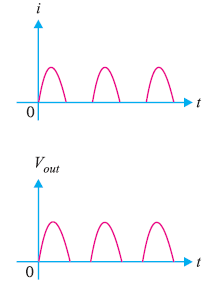Fig(2)

Fig(1) shows the circuit connection of a half wave rectifier .

Fig(2) shows the input and output waveform of a half wave rectifier .

In this circuit a single crystal diode is used  as a half wave rectifier .

The a.c. supply is applied in series with the diode D and load resistance RL

The a.c. supply is normally applied through a transformer. It gives two advantages. First it allows us to step up or step down the a.c. input voltage as required. Secondly, the transformer isolates the rectifier circuit from power line and thus reduces the risk of electric shock.

### Operation of Half  wave Rectifier

During the positive half cycles of input supply voltage, end A becomes positive with respect to end B.

This makes the diode D forward biased and hence it conducts current.

During negative half cycles, end A becomes negative with respect to end B. So the diode D is reverse biased and hence conducts no current.

So current flows through the diode only during the positive half cycles of the input a.c. voltage.

Hence current flows through the load Ralways in the same direction and d.c. output is obtained across RL

In half wave rectifier , output across the load is pulsating. So a filter circuit is used to smoothen the  output.

### Disadvantages of Half Wave Rectifier

The output is pulsating, so a filter circuit is required.

The a.c. supply delivers power only half the time. So output is low.

### Output Frequency

The output frequency of a half wave rectifier is equal to the input frequency i.e 50 Hz.

fout = fin

### Efficiency

The ratio of d.c. power output to the applied input a.c. power is known as rectifier efficiency.

Rectifier efficiency

Let the input supply voltage which appears across the secondary winding

v  =  Vsinθ

Diode resistance = rf

The diode conducts only during the positive half cycles of the a.c. supply.

#### D.C. Power Output

The output current is pulsating direct current.

Iav=Idc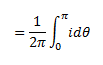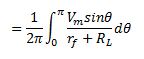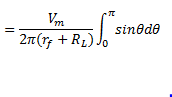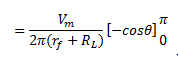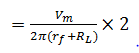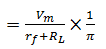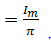D.C. power,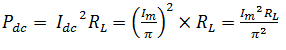#### A.C. Power input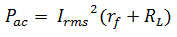### Rectifier Efficiency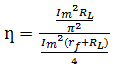η will be maximum if  rf is  negligible as compared to RL

Hence maximum efficiency = 40.6%

This means in Half wave rectifier , a maximum of 40.6% of a.c. power is converted into d.c. power.

### Ripple Factor

In half-wave rectification,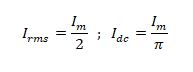hence,Hence it is clear that a.c. component exceeds the d.c. component in the output of a half wave rectifier .It results more pulsation in the output. So half wave rectifier is ineffective for conversion of a.c into d.c.